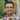# Fun with Hexagon Math for Games

Hexagons are great for game grids because, compared to the standard square grid, the distance from the center of a hex to any adjacent hex is the same (assuming you have a “Normal” hex with width to height ratio of 2:√3). In a square grid, moving from one square to an adjacent diagonal square is further away then moving horizontally or vertically to an adjacent square.

The mathematics and programming of Hexagons for a game grid can get a bit more complex than the math and programming for squares. You have to create polygons instead of rectangles, when you create a grid of hexagons you have to offset each row by half the width of the hex, pathfinding can be more complex, and hit detection is more complex.

There are some choices you have to make when creating your hex grid: How big are my hexagons going to be? Are my hexagons going to be symmetric? Meaning the width is equal to the height (ratio 1:1, or square). Or will I choose some other ratio of width to height for my hexagons? A “Regular Hexagon” is quite common since all the angles and side lengths are equal (consequently the width to height ratio of regular hexagons turns out to be 2/√3).

The key variables we need to know before we are ready to draw a hexagon are: Width (w), Height (h), and side length (z). We will also define x and y to be the other sides of the triangle made using the top left angled side as shown in this image:#### Width and Height are known

Let’s say we know we want our hexes to be a certain size, so we know the width and height (maybe 100x100), but we also need to know z, the length of each side.

We know from the Pythagorean theorem that we have:

$x^2 + y^2 = z^2$

Also, from the above image you can see that there is a relationship between x, z and the width, and y and the height, specifically:

$2x + z = w$

and

$2y = h$

Now we should be able to solve for z (our side length).

$x = \frac{w - z}{2}$ $y = \frac{h}{2}$

Plugging x and y back into pythagoras we get:

$(\frac{w - z}{2})^2 + (\frac{h}{2})^2 = z^2$

After expanding and simplifying:

$-3z^2 - 2wz + h^2 + w^2 = 0$

Remember that w and h are constants we have already chosen, so we can solve this using the quadratic formula:

$x=\frac{-b \pm \sqrt {b^2-4ac}}{2a}$

where

$ax^2 + bx + c = 0$

In our case:

$a = -3$ $b = -2w$ $c = h^2 + w^2$

Thus, to get z (the hex side length from the width and height we can use this formula:

$z=\frac{2w - \sqrt {4w^2+12(h^2+w^2)}}{-6}$

#### Side Length and Ratio are known

Now let’s say instead of knowing the width and height for our hexagons, we know we want a certain side length z (say 100) and a certain width to height ratio r (say of 4:3, or 1.3).

We can still use our formulas from above, pythagoras and our formulas relating x, y and z to the width and height. Now since we don’t know the exact width and height, we’ll substitute that ratio (r) variable for w and h:

$r = \frac{w}{h}$

And using our equations from above:

$r = \frac{2x + z}{2y}$

Solving for y:

$y = \frac{2x + z}{2r}$

Now we’ll substitute our value for y into pythagoras:

$x^2 + (\frac{2x + z}{2r})^2 = z^2$

After simplifying and turning this into quadratic form:

$(\frac{1+r^2}{r^2})x^2 + \frac{z}{r^2}x + (\frac{1-4r^2}{4r^2})z^2 = 0$

Now for the quadratic we have:

$a = \frac{1+r^2}{r^2}$ $b = \frac{z}{r^2}$ $c = (\frac{1-4r^2}{4r^2})z^2$

If we substitute this into the quadratic, it looks pretty ugly:

$x=\frac{-(\frac{z}{r^2}) + \sqrt {(\frac{z}{r^2})^2-4(\frac{1+r^2}{r^2})(\frac{1-4r^2}{4r^2})z^2}}{2(\frac{1+r^2}{r^2})}$

#### Other Great Hex for Game Programming Resources:

Amit Patel’s Thoughts on Grids PlayTechs: Programming for fun Hex Grids Hexnet.org’s Introduction to Hexagonal Geometry

#### Can you do this for me? My HTML5 Hexagon Tools

If you are like me, you skim over all these equations and just want to get to the good stuff, like concrete examples. So I’ve put together a simple form to do these calculations for you using JavaScript. Once you fill out the form you should see a visual representation of the computed hexagon, drawn on a canvas (assuming you have an HTML5 enabled browser).

#### Source Files

If you want to take a look at the JavasScript for this here are my source files, you are welcome to use these for your own projects: HexCalcs.js HexagonTools.js

HexCalcs.js is mostly just for parsing the forms above and performing the math described in this post. HexagonTools.js defines a Hexagon class that defines all the points in a hexagon and defines a draw method for the canvas.

You can also find the entire HexagonTools library code on GitHub.

In a future post I’ll show how to use the Hexagon class to build a Hex grid.